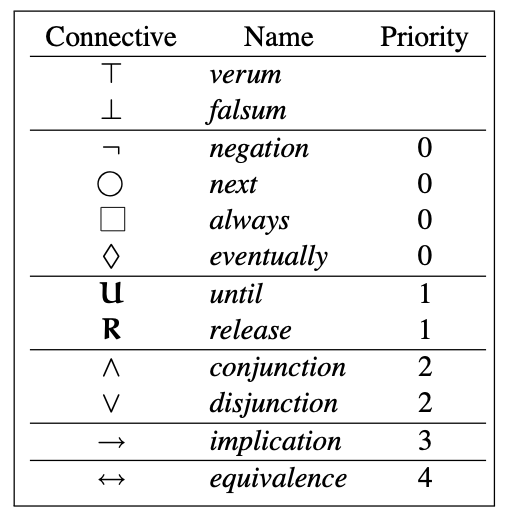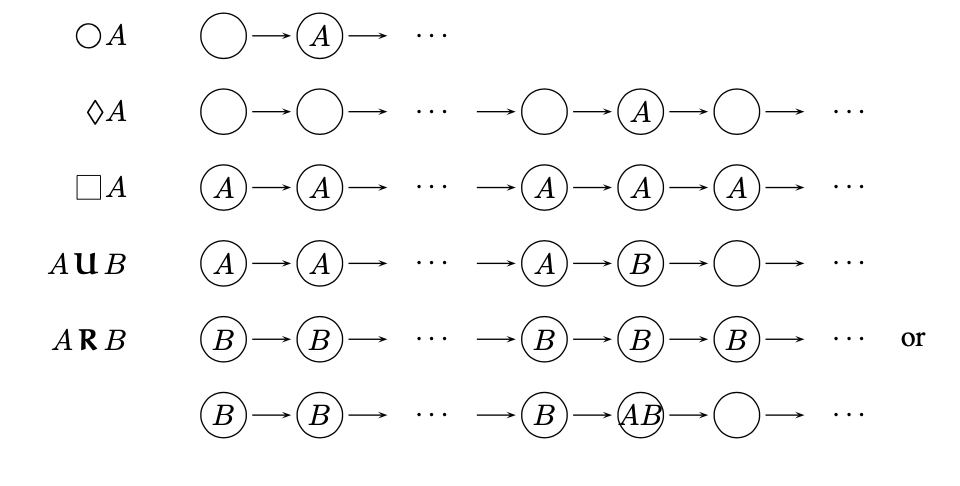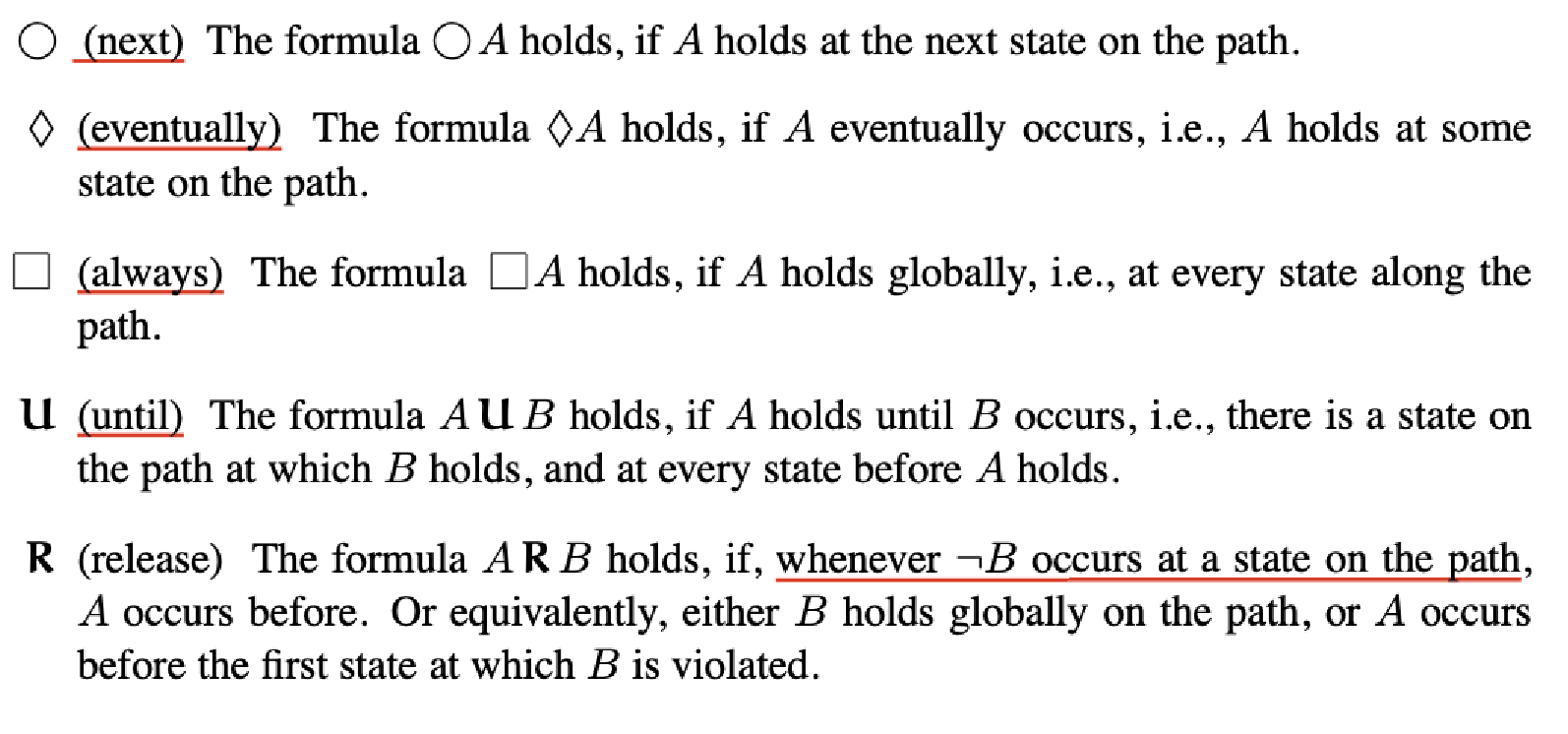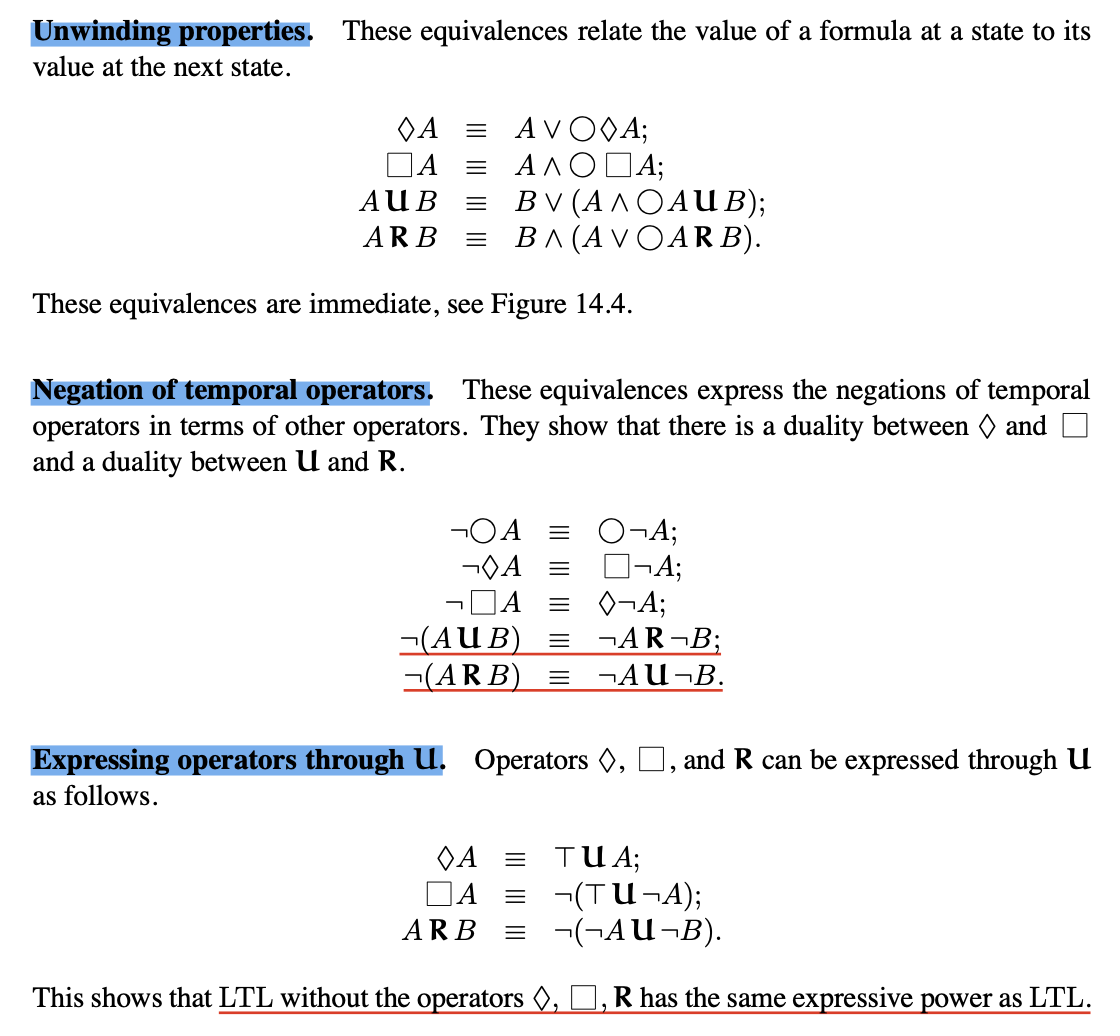# 逻辑学探幽 Part12

## 没有逻辑 只有heip

Posted by R1NG on December 16, 2021 Viewed Times

# 线性时态逻辑

## 12.1 计算树

$\mathbb{S} = (S, \text{In}, T, \mathscr{X}, \text{dom}, L),$

1. 计算树的节点由 $S$ 中的状态所标记.
2. 树的根节点由 $s$ 所标记.
3. 对树中的每个节点 $s’$, 其任一子节点 $s’’$ 恰满足条件 $(s’, s’’) \in T$.

1. 对任意 $i \in [n-1]$, 有 $(s_i, s_{i+1})\in T$.
2. 若该序列是有限的, 则不存在任何 $s$ 满足 $(s_n, s) \in T$.

1. 某个状态转换系统的计算路径恰为该状态转换系统计算树中的所有分支.
2. 任何计算树的子树也是一棵计算树.
3. 对任何状态转换系统 $\mathbb{S}$ 和状态 $s$, 一定唯一存在某个 $\mathbb{S}$ 中以 $s$ 为根节点的计算树.

## 12.2 线性时态逻辑

1. $\top$ 和 $\perp$ 均被视为公式.
2. PLFD 中的任何原子公式 (形如 $x=v$) 被视为 LTL 中的原子公式.
3. 若 $A_1, \cdots, A_n$ 为公式, 其中 $n \geqslant 2$, 则 $(A_1 \wedge \cdots \wedge A_n)$ 和 $(A_1 \vee \cdots \vee A_n)$ 也都是公式.
4. 若 $A$ 为公式, 则 $\neg A$ 也是公式.
5. 若 $A$ 和 $B$ 均为公式, 则 $(A \rightarrow B)$ 和 $(A \leftrightarrow B)$ 也都是公式.
6. 若 $A$ 为公式, 则 $\bigcirc$, $\lozenge$, $\square$ 均为公式.LTL (线性时态逻辑) 中的公式的真伪性都是在定义在某个计算路径上的. 举例而言:

1. 公式 $\square ~ A$ 表明, $A$ 在计算路径上 恒为真.
2. 公式 $\lozenge ~A$ 表明, $A$ 在计算路径的 某一个状态上 为真.
3. 公式 $\bigcirc ~A$ 表明, $A$ 在计算路径的初始状态 $s_0$ 的下一个状态 $s_1$ 上为真.

1. $\pi \vDash \top$ 且 $\pi \nvDash \perp$.
2. $\pi \vDash x=v$ 若 $s_0 \vDash x=v$.
3. $\pi \vDash A_1 \wedge \cdots \wedge A_n$ 若对任意 $j \in [n]$, 有 $\pi \vDash A_j$.
4. $\pi \vDash A_1 \vee \cdots \vee A_n$ 若对任意 $j \in [n]$, 至少有一个 $j$ 满足 $\pi \vDash A_j$.
5. $\pi \vDash \neg A$ 若 $\pi \nvDash A$.
6. $\pi \vDash \bigcirc ~A$ 若 $\pi \vDash A$.
7. $\pi \vDash \lozenge ~A$ 若对某个 $i \in [n]$, 满足 $\pi_i \vDash A$.
8. $\pi \vDash \square ~A$ 若对任意 $i \in [n]$, 满足 $\pi_i \vDash A$.
9. $\pi \vDash A~\mathcal{U}~B$ 若对某个 $k \in [n]$, 我们有 $p_k \vDash B$ 且 $p_0 \vDash A, \cdots, p_{k-1} \vDash A$.
10. $\pi \vDash A~\mathcal{R}~B$ 若对任意 $k \in [n]$, 要么 $\pi_k \vDash B$, 要么存在 $j < k$ 使得 $\pi_j \vDash A$.

1. 线性时态逻辑中的公式 $A, B$ 是等价 (记为 $A \equiv B$) 的, 当且仅当对计算树中的 任何 计算路径 $\pi$, $\pi \vDash A$ 当且仅当 $\pi \vDash B$.

2. 我们一般将 “线性时态逻辑公式 $A$ 在路径 $\pi_i$ 上为真” 简称为 “线性时态逻辑公式 $A$ 在状态 $s_i$ 上为真”.## 12.3 时态公式的等价性• Every Computation Tree is a binary tree – FALSE
• If an LTL formula is true on a path then its negation is false on this path. – TRUE
• If an LTL formula is true on all paths then its negation is false on all paths. – TRUE
• If a formula F does not contain temporal operators then F is true on a path s_0,s_1,..,if and only if F is true in s_0. – TRUE
• For every LTL formula there is an equivalent formula that does not contain operators [] and <>. – TRUE